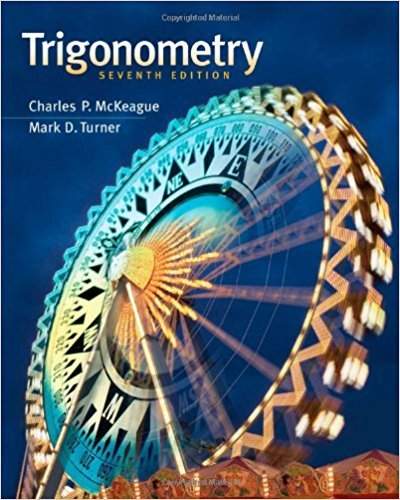×
×

# Solutions for Chapter 1-22: Complex Numbers and Polar Coordinates## Full solutions for Trigonometry | 7th Edition

ISBN: 9781111826857Solutions for Chapter 1-22: Complex Numbers and Polar Coordinates

Solutions for Chapter 1-22
4 5 0 328 Reviews
18
4
##### ISBN: 9781111826857

Trigonometry was written by and is associated to the ISBN: 9781111826857. This expansive textbook survival guide covers the following chapters and their solutions. Since 1 problems in chapter 1-22: Complex Numbers and Polar Coordinates have been answered, more than 26282 students have viewed full step-by-step solutions from this chapter. This textbook survival guide was created for the textbook: Trigonometry, edition: 7. Chapter 1-22: Complex Numbers and Polar Coordinates includes 1 full step-by-step solutions.

Key Math Terms and definitions covered in this textbook
• Cross product u xv in R3:

Vector perpendicular to u and v, length Ilullllvlll sin el = area of parallelogram, u x v = "determinant" of [i j k; UI U2 U3; VI V2 V3].

• Determinant IAI = det(A).

Defined by det I = 1, sign reversal for row exchange, and linearity in each row. Then IAI = 0 when A is singular. Also IABI = IAIIBI and

• Independent vectors VI, .. " vk.

No combination cl VI + ... + qVk = zero vector unless all ci = O. If the v's are the columns of A, the only solution to Ax = 0 is x = o.

• Iterative method.

A sequence of steps intended to approach the desired solution.

• Kronecker product (tensor product) A ® B.

Blocks aij B, eigenvalues Ap(A)Aq(B).

• Left inverse A+.

If A has full column rank n, then A+ = (AT A)-I AT has A+ A = In.

• Nilpotent matrix N.

Some power of N is the zero matrix, N k = o. The only eigenvalue is A = 0 (repeated n times). Examples: triangular matrices with zero diagonal.

• Orthogonal matrix Q.

Square matrix with orthonormal columns, so QT = Q-l. Preserves length and angles, IIQxll = IIxll and (QX)T(Qy) = xTy. AlllAI = 1, with orthogonal eigenvectors. Examples: Rotation, reflection, permutation.

• Pseudoinverse A+ (Moore-Penrose inverse).

The n by m matrix that "inverts" A from column space back to row space, with N(A+) = N(AT). A+ A and AA+ are the projection matrices onto the row space and column space. Rank(A +) = rank(A).

• Rank r (A)

= number of pivots = dimension of column space = dimension of row space.

• Saddle point of I(x}, ... ,xn ).

A point where the first derivatives of I are zero and the second derivative matrix (a2 II aXi ax j = Hessian matrix) is indefinite.

• Schur complement S, D - C A -} B.

Appears in block elimination on [~ g ].

• Skew-symmetric matrix K.

The transpose is -K, since Kij = -Kji. Eigenvalues are pure imaginary, eigenvectors are orthogonal, eKt is an orthogonal matrix.

• Spanning set.

Combinations of VI, ... ,Vm fill the space. The columns of A span C (A)!

• Sum V + W of subs paces.

Space of all (v in V) + (w in W). Direct sum: V n W = to}.

• Symmetric matrix A.

The transpose is AT = A, and aU = a ji. A-I is also symmetric.

• Toeplitz matrix.

Constant down each diagonal = time-invariant (shift-invariant) filter.

• Transpose matrix AT.

Entries AL = Ajj. AT is n by In, AT A is square, symmetric, positive semidefinite. The transposes of AB and A-I are BT AT and (AT)-I.

• Unitary matrix UH = U T = U-I.

Orthonormal columns (complex analog of Q).